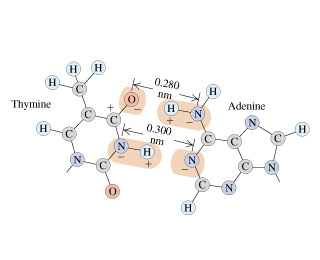Question
1285 views

The two sides of the DNA double helix are connected by pairs of bases (adenine, thymine, cytosine, and guanine). Because of the geometric shape of these molecules, adenine bonds with thymine and cytosine bonds with guanine. The figure (Figure 1) shows the thymine-adenine bond. Each charge shown is ±e, and the H−N distance is 0.110 nm.

A) Calculate the net force that thymine exerts on adenine. To keep the calculations fairly simple, yet reasonable, consider only the forces due to the O−H−N and the N−H−N combinations, assuming that these two combinations are parallel to each other. Remember, however, that in the O−H−N set, the O− exerts a force on both the H+ and the N−, and likewise along the N−H−N set.

B) Is the net force attractive or repulsive?

C) Calculate the force on the electron in the hydrogen atom, which is 5.29×10−2 nm from the proton.

D) Compare the strength of the bonding force of the electron in hydrogen with the bonding force of the adenine-thymine molecules.help_outlineImage Transcriptionclose(н н 0.280 (н) н nm н Adenine N Thymine 0.300 н nm N. N N fullscreen
check_circle

Step 1

The force exerted by O- on H+ and N- has to be calculated.

This is a force of repulsion between O- and N-.

Step 2

Net force on H-N by O is:

Step 3

Force exerted by O- on H+ and N- is 502.79×10-11N towards thymine.

There is a force of repulsion betw...

### Want to see the full answer?

See Solution

#### Want to see this answer and more?

Solutions are written by subject experts who are available 24/7. Questions are typically answered within 1 hour.*

See Solution
*Response times may vary by subject and question.
Tagged in

### Chemistry